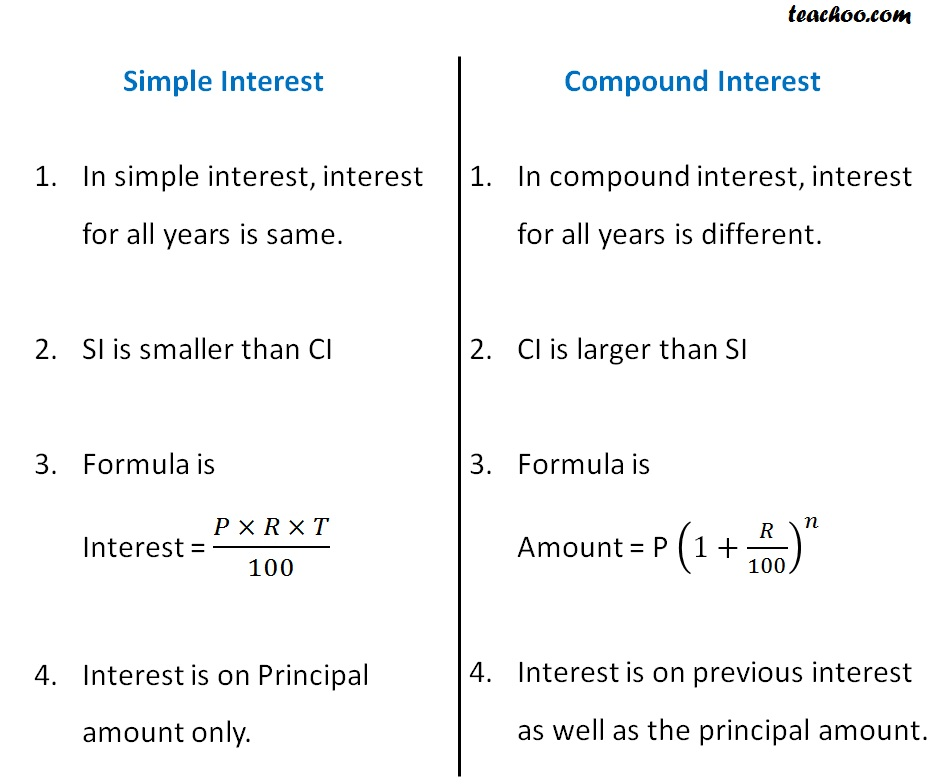Compound Interest

Chapter 7 Class 8 Comparing Quantities
Concept wiseLearn in your speed, with individual attention - Teachoo Maths 1-on-1 Class

### Transcript

Difference between Simple Interest & Compound Interest Simple Interest In simple interest, interest for all years is same. SI is smaller than CI Formula is Interest = (𝑃 × 𝑅 × 𝑇)/100 Interest is on Principal amount only. Compound Interest In compound interest, interest for all years is different. CI is larger than SI Formula is Amount = P (1+𝑅/100)^𝑛 Interest is on previous interest as well as the principal amount.